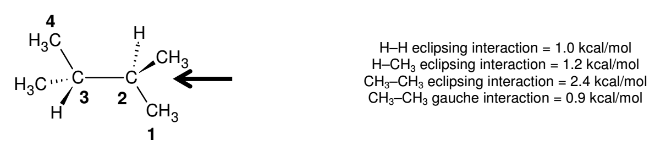# Problem: What is the total strain energy (in kcal/mol) of the specific conformation of 2, 3–dimethylbutane shown below? All of the choices below are expressed in units of kcal/mol.   A. 0          B. 0.9          C. 1.8          D. 2.7         E. 3.4 F. 3.6       G. 4.4          H. 5.8           I. 7.2          J. None of these

###### Problem Details

What is the total strain energy (in kcal/mol) of the specific conformation of 2, 3–dimethylbutane shown below? All of the choices below are expressed in units of kcal/mol.

A. 0          B. 0.9          C. 1.8          D. 2.7         E. 3.4

F. 3.6       G. 4.4          H. 5.8           I. 7.2          J. None of these